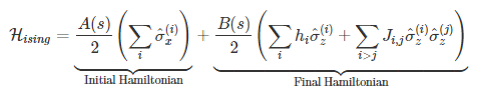# What Is the Hamiltonian?

A classical Hamiltonian is a mathematical description of some physical system in terms of its energies. We can input any particular state of the system, and the Hamiltonian returns the energy for that state. For most non-convex Hamiltonians, finding the minimum energy state is an NP-hard problem that classical computers cannot solve efficiently.

As an example of a classical system, consider an extremely simple system of a table and an apple. This system has two possible states: the apple on the table, and the apple on the floor. The Hamiltonian tells us the energies, from which we can discern that the state with the apple on the table has a higher energy than that when the apple is on the floor.

For the D-Wave system, the Hamiltonian may be represented aswhere σ are Pauli matrices operating on a qubit q, and h and J are the qubit biases and coupling strengths.  A(s) and B(s) are energy scaling functions which evolve according to the anneal fraction, s.

For further information on the Hamiltonian, watch the video below, and read the Getting Started guide on the D-Wave Documentation site.